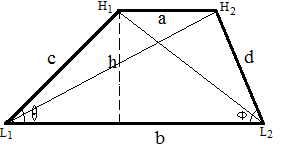梯形在线计算器

 平行边a： 平行边b： 角 (θ)： 弧度  ̊ 度  π  π/3 角 (Φ)： 弧度  ̊ 度  π  π/3 高 (h)： 面积： 周长： 边 c： 边 d： 对角线 L1H2： 对角线 H1L2：

梯形公式1. 面积 = h(a+b)/2

2. 周长 = a + b + c + d

3. 对角线 L1H2 = sqrt(h2+(b-sqrt(c2-h2))2)

4. 对角线 H1L2 = sqrt(h2+(b-sqrt(d2-h2))2)